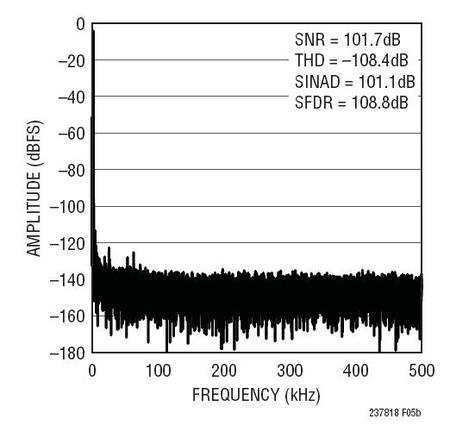# ADC Driving: Single-Ended To Differential Conversion

Figure 1 shows the LT6350 being used to convert a 0V to 5V single-ended input signal to differential for an ADC with differential inputs. In this case, the first amplifier is configured as a unity gain buffer and the single-ended input signal directly drives the high-impedance input of the amplifier. As shown in the FFT of Figure 2, the LT6350 drives the LTC2378-18 to near full datasheet performance.Figure 1. LT6350 Converting a 0V to 5V Single Ended Signal to a ±5V Differential SignalFigure 2. 32k Point FFT Plot with Fin=2kHz for Circuit Shown in Figure 1

Figure 3 shows the LT6350 being used to convert a ±10V true bipolar signal for use by the LTC2378-18. In this case, the first amplifier in the LT6350 is configured as an inverting amplifier stage, which acts to attenuate and level shift the input signal to the 0V to 5V input range of the LTC2378-18. In the inverting amplifier configuration, the single-ended input signal source no longer directly drives a high impedance input of the first amplifier. The input impedance is instead set by resistor RIN. RIN must be chosen carefully based on the source impedance of the signal source. Higher values of RIN tend to degrade both the noise and distortion of the LT6350 and LTC2378-18 as a system.  R1, R2, R3 and R4 must be selected in relation to RIN to achieve the desired attenuation and to maintain a balanced input impedance in the first amplifier. Table 1 shows the resulting SNR and THD for several values of RIN, R1, R2, R3 and R4 in this configuration. Figure 4 shows the resulting FFT when using the LT6350 as shown in Figure 3.Figure 3. LT6350 Converting a ±10V Single-Ended Signal to a ±5V Differential SignalFigure 4. 32k Point FFT Plot with Fin=2kHz for Circuit Shown in Figure 3Table 1. SNR, THD vs. Rin for ±10V Single-Ended Input Signal

Figure 5 shows how to configure the LT6350 to accept a ±10V true bipolar input signal and attenuate and level shift the signal to the reduced input range of the LTC2378-18 when digital gain compression is enabled. Figure 6 shows an FFT plot with the LTC2378-18 being driven by the LT6350 with digital gain compression enabled.Figure 5. LT6350 Configured to Accept a ±10V Input Signal While Running Off of a Single 5.5V Supply When Digital Gain Compression Is Enabled in the LTC2378-18Figure 6. 32k Point FFT Plot with Fin=2kHz for Circuit Shown in Figure 5